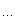# coreBugZJ

## 归并树

1 // MergeTree begin
2
3 // T(),  T < T, T = T
4 // ensure 2^NL >  ri
5 // [ le, ri ]
6
7 template<class T, int NL>
8 class MergeTree
9 {
10 public :
11         void init( const T d[], int le, int ri, int dep = 0int v = 1 ) {
12                 left[ v ]  = le;
13                 right[ v ] = ri;
14                 if ( le == ri ) {
15                         data[ dep ][ le ] = d[ le ];
16                         return;
17                 }
18                 int mid = ( le + ri ) / 2;
19                 init( d, le, mid, dep + 1, v + v );
20                 init( d, mid + 1, ri, dep + 1, v + v + 1 );
21                 int i = le, j = mid + 1, k = le;
22                 while ( ( i <= mid ) && ( j <= ri ) ) {
23                         if ( data[ dep + 1 ][ i ] < data[ dep + 1 ][ j ] ) {
24                                 data[ dep ][ k++ ] = data[ dep + 1 ][ i++ ];
25                         }
26                         else {
27                                 data[ dep ][ k++ ] = data[ dep + 1 ][ j++ ];
28                         }
29                 }
30                 while ( i <= mid ) {
31                         data[ dep ][ k++ ] = data[ dep + 1 ][ i++ ];
32                 }
33                 while ( j <= ri ) {
34                         data[ dep ][ k++ ] = data[ dep + 1 ][ j++ ];
35                 }
36         }
37         int getCountBelow( int le, int ri, const T & da, int dep = 0int v = 1 ) {
38                 if ( ( ri < left[ v ] ) || ( right[ v ] < le ) ) {
39                         return 0;
40                 }
41                 if ( ( le <= left[ v ] ) && ( right[ v ] <= ri ) ) {
42                         int low = left[ v ], high = right[ v ], mid, ans = left[ v ] - 1;
43                         while ( low <= high ) {
44                                 mid = ( low + high ) >> 1;
45                                 if ( data[ dep ][ mid ] < da ) {
46                                         low = mid + 1;
47                                         if ( ans < mid ) {
48                                                 ans = mid;
49                                         }
50                                 }
51                                 else {
52                                         high = mid - 1;
53                                 }
54                         }
55                         return ans - left[ v ] + 1;
56                 }
57                 return getCountBelow( le, ri, da, dep + 1, v + v ) +
58                        getCountBelow( le, ri, da, dep + 1, v + v + 1 );
59         }
60         // k = 1, 2,, ri - le + 1, le <= ri
61         T getKth( int le, int ri, int k ) {
62                 int low = left[ 1 ], high = right[ 1 ], mid, rk, ans = left[ 1 ];
63                 while ( low <= high ) {
64                         mid = ( low + high ) >> 1;
65                         rk = getCountBelow( le, ri, data[ 0 ][ mid ] ) + 1;
66                         if ( rk <= k ) {
67                                 low = mid + 1;
68                                 if ( mid > ans ) {
69                                         ans = mid;
70                                 }
71                         }
72                         else {
73                                 high = mid - 1;
74                         }
75                 }
76                 return data[ 0 ][ ans ];
77         }
78 private :
79         T  data[ NL ][ 1 << NL ];
80         int left[ 4 << NL ], right[ 4 << NL ];
81 };
82
83 // MergeTree end
84

posted on 2011-03-20 19:44 coreBugZJ 阅读(1695) 评论(0)  编辑 收藏 引用 所属分类: DataStructure